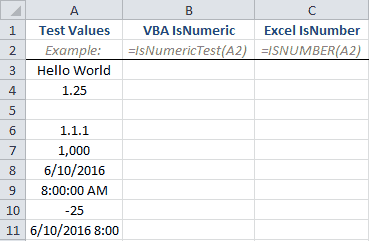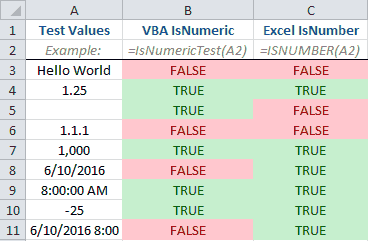## Introduction to IsNumeric

The IsNumeric VBA function checks if a cell is a number and expresses the answer as a Logical Boolean (`True` or `False`). The IsNumeric VBA function is a counterpart to the Excel ISNUMBER function, but the expressions don’t always produce the same results.

This isn’t the first time you’ve seen me use IsNumeric in my VBA macros, but in this tutorial I’ll explain how it’s used and what it’s good for. Let’s get started.

• If IsNumeric thinks your expression is a number, it will return a value of True.
• If it’s not a number, it will return False.

The syntax for the IsNumeric function can’t get any simpler:

`IsNumeric(Expression)`

As you can see, IsNumeric accepts one argument: an expression of the Variant Data Type. I know, I know. The complicated jargon isn’t necessary.

All you need to know is that IsNumeric can pretty much evaluate anything. A cell, a string, a date, a range. It won’t choke up on any of these, but that doesn’t mean it’ll give you the answer you want.

For example, if you enter a range, like Range("A1:B5"), into an IsNumeric expression, it will always return False even if all the values in the range ARE numeric. The IsNumeric function won’t loop through each cell in your range and check whether each of them are numeric. You’ll have to do that with a loop of your own, like a For Each loop. I’ll show you a macro that does that in the IsNumeric Examples section.

Because IsNumeric returns True or False, it’s a great expression to include inside If Statements. Let’s take a look at a couple examples.

## IsNumeric Examples

### Check if a Cell is a Number

``````Sub IsNumericDemo()
'Use VBA to test if a cell is numeric via a subroutine
If IsNumeric(Range("A1")) = True Then
Range("B1") = "A1 is a number"
Else
Range("B1") = "A1 is not number"
End If
End Sub``````

Make powerful macros with our free VBA Developer Kit

It’s easy to copy and paste a macro like this, but it’s harder make one on your own. To help you make macros like this, we built a free VBA Developer Kit and wrote the Big Book of Excel VBA Macros full of hundreds of pre-built macros to help you master file I/O, arrays, strings and more - grab your free copy below.

I'll take a free VBA Developer Kit

This example macro tests if the value in cell A1 is a number. If it is, the value in cell B1 says it’s a number. Otherwise, it says it’s not a number.

You don’t need to put the `= True` in the above If Statement, but I included it to make the macro easier to read.

If you have a macro that performs arithmetic expressions, it’s a good practice to use IsNumeric to make sure your input is numeric before performing the math. As a side note, it’s also a good to make sure your input isn’t empty by using the `IsEmpty` function. That’s another tutorial for another day.

### Check if All Cells in a Range are Numeric

``````Sub IsNumericRange()
Dim cell As Range
Dim bIsNumeric As Boolean

bIsNumeric = True
For Each cell In Range("A1:B5")
If IsNumeric(cell) = False Then
'Non-numeric value found. Exit loop
bIsNumeric = False
Exit For
End If
Next cell

If bIsNumeric = True Then
'All values in your range are numeric
'**PLACE CODE HERE**
MsgBox "All values are numeric"
Else
'There are non-numeric values in your range
'**PLACE CODE HERE**
MsgBox "There are non-numeric values in your range"
End If
End Sub``````

The above macro checks each cell in your range and the moment it finds one that isn’t numeric, it exits the For Each loop and lets you know there are non-numeric cells in the range.

As a programmer, you can perform different actions based on whether the entire range is numeric or not. Checks like this one give you more control over how you handle errors.

## IsNumeric vs ISNUMBER

To test how the IsNumeric VBA and the ISNUMBER Excel functions behave, we’re going to make a User Defined Function (UDF) to evaluate the following cells in Column A:We’ll use the native ISNUMBER function of Excel in Column C and we’ll use the the following UDF to represent our VBA IsNumeric function in Column B.

``````Function IsNumericTest(TestCell As Variant)
'Use VBA to test if a cell is numeric via a function
If IsNumeric(TestCell) Then 'if TestCell is True
IsNumericTest = True 'Cell is a number
Else
IsNumericTest = False 'Cell is not a number
End If
End Function``````

We’ll evalulate the expressions in Column A using both the VBA `IsNumeric()` function (Column B) and the Excel `=ISNUMBER()` function (Column C). You would expect them to be identical, right? You’re about to be surprised…The two functions yield completely different answers when evaluating the same data. IT’S CRAZY!

By looking at the comparison image, you can see the VBA IsNumeric function considers empty cells numeric, but the Excel ISNUMBER function does not. That’s why I said earlier that it’s a good VBA practice to check if your cell is empty by using the `IsEmpty` function when you use the IsNumeric function.

Another difference you can see is in how the two functions treat dates and times. IsNumeric VBA says times are numbers, but dates are not. It also says the combination of dates and times are not numeric. ISNUMBER, on the other hand, says all 3 date/time cells are numeric.

## Final Thoughts

Congratulations! You’re now an IsNumeric expert! You certainly know more about IsNumeric than the average Excel user, and for that you should be excited.

IsNumeric is a great VBA function, but, as you’ve seen, you have to be careful when using it if there’s a chance your input may be blank or if you’re evaluating dates and times.

It’s important to know what a function does well, but it’s equally important to know what a function doesn’t do well. I hope you find this VBA tutorial informative and you’re IsNumeric in your own macros!

For more VBA tips, techniques, and tactics, subscribe to our VBA Insiders email series using the form below. After you subscribe, share what you’re automating on Twitter and Facebook.